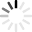# Polynomial Quizzes & Trivia

Can you factor the polynomial 4x^2-25 using the difference of two perfect squares? Do you have the math skills necessary to completely factor 2x^2-50? Take the online polynomial quizzes and give your polynomial factoring skills a touch-up.
Top Trending

This quiz is all about polynomial function, 1-30 items multiple choice. This will help you become a better learner in the basics and fundamentals of algebra.

Questions: 30  |  Attempts: 3950   |  Last updated: Nov 5, 2020
• Sample Question
What is the leading term of  ?This quiz aims to let the student find the degree of each given polynomial. This can be given to Grade Six or First Year High School Students.

Questions: 10  |  Attempts: 4686   |  Last updated: Jan 23, 2013

Are you ready to test your knowledge of polynomial division? Do you know all there is to know about synthetic division? Get ready to test your knowledge! NOTE: You will probably want to have a piece of scrap paper and a pencil...

Questions: 12  |  Attempts: 810   |  Last updated: Dec 26, 2012
• Sample Question
Which of the following is a polynomial term?Questions: 10  |  Attempts: 341   |  Last updated: Feb 23, 2018
• Sample Question
(-x3 + 3x2 + 3) + (3x2 + x + 4) A. -x3 + 6x2 + x + 7 B. -x3 + 9x2 + x + 7 C. 2x6 + x + 7 D. 2x5 - x + 7Complete the following questions

Questions: 9  |  Attempts: 389   |  Last updated: Jan 7, 2013
• Sample Question
What is the greatest common factor of the polynomial 8x+14x–32?TopicsRelated